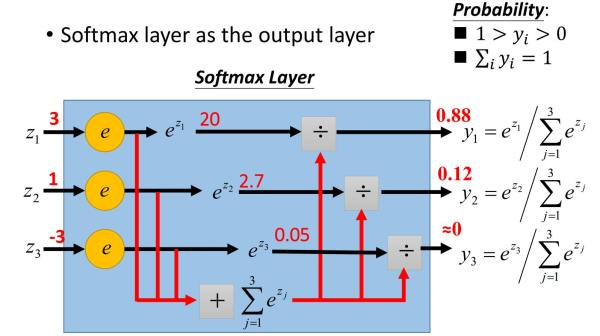## [原创] 如何防止softmax函数上溢出(overflow)和下溢出(underflow)

Deep Learning》（Ian Goodfellow & Yoshua Bengio & Aaron Courville）第四章「数值计算」中，谈到了上溢出（overflow）和下溢出（underflow）对数值计算的影响，并以softmax函数和log softmax函数为例进行了讲解。这里我再详细地把它总结一下。

『1』什么是下溢出（underflow）和上溢出（overflow）

『2』softmax函数是什么
softmax函数如下：
$f{(x)_i} = \frac{{{e^{{x_i}}}}}{{\sum\limits_{j = 1}^n {{e^{{x_j}}}} }},j = 1,2,...,n$『2』计算softmax函数值的问题

• c 极其大，导致分子计算 ${e^c}$ 时上溢出
• c 为负数，且  $\left| c \right|$ 很大，此时分母是一个极小的正数，有可能四舍五入为0，导致下溢出

『3』如何解决

$\frac{{{e^{{z_2}}}}}{{{e^{{z_1}}} + {e^{{z_2}}} + {e^{{z_3}}}}} = \frac{{{e^1}}}{{{e^3} + {e^1} + {e^{ - 3}}}} = \frac{{2.7}}{{20 + 2.7 + 0.05}} \approx 0.12$

$\frac{{{e^{({z_2} - M)}}}}{{{e^{({z_1} - M)}} + {e^{({z_2} - M)}} + {e^{({z_3} - M)}}}} = \frac{{{e^{(1 - 3)}}}}{{{e^{(3 - 3)}} + {e^{(1 - 3)}} + {e^{( - 3 - 3)}}}} \approx 0.12$

$\frac{{{e^{{z_2}}}}}{{{e^{{z_1}}} + {e^{{z_2}}} + {e^{{z_3}}}}} = \frac{{\frac{{{e^{{z_2}}}}}{{{e^M}}}}}{{\frac{{{e^{{z_1}}} + {e^{{z_2}}} + {e^{{z_3}}}}}{{{e^M}}}}} = \frac{{\frac{{{e^{{z_2}}}}}{{{e^M}}}}}{{\frac{{{e^{{z_1}}}}}{{{e^M}}} + \frac{{{e^{{z_2}}}}}{{{e^M}}} + \frac{{{e^{{z_3}}}}}{{{e^M}}}}} = \frac{{{e^{\left( {{z_2} - M} \right)}}}}{{{e^{\left( {{z_1} - M} \right)}} + {e^{\left( {{z_2} - M} \right)}} + {e^{\left( {{z_3} - M} \right)}}}}$

『4』延伸问题

$\log [f({x_i})] = \log \left( {\frac{{{e^{{x_i}}}}}{{{e^{{x_1}}} + {e^{{x_2}}} + \cdots {e^{{x_n}}}}}} \right) = \log \left( {\frac{{\frac{{{e^{{x_i}}}}}{{{e^M}}}}}{{\frac{{{e^{{x_1}}}}}{{{e^M}}} + \frac{{{e^{{x_2}}}}}{{{e^M}}} + \cdots \frac{{{e^{{x_n}}}}}{{{e^M}}}}}} \right) = \log \left( {\frac{{{e^{\left( {{x_i} - M} \right)}}}}{{\sum\limits_j^n {{e^{\left( {{x_j} - M} \right)}}} }}} \right) = \log \left( {{e^{\left( {{x_i} - M} \right)}}} \right) - \log \left( {\sum\limits_j^n {{e^{\left( {{x_j} - M} \right)}}} } \right) = \left( {{x_i} - M} \right) - \log \left( {\sum\limits_j^n {{e^{\left( {{x_j} - M} \right)}}} } \right)$

➤➤ 版权声明 ➤➤## One thought on “[原创] 如何防止softmax函数上溢出(overflow)和下溢出(underflow)”

1. 姓名说道：

非常有用，博主不错，加油

2. Yunlei Zhang说道：

此时分子是一个极小的正数

3. Yunlei Zhang说道：

看似已经结案了，但仍然有一个问题：如果softmax函数中的分子发生下溢出，也就是前面所说的 c 为负数，且 |c| 很大，此时分母是一个极小的正数。。。这句话中，应该是“此时分子是一个极小的正数”